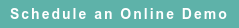# Blog

### Posts by Topic

We must be careful when the profit rate (“return on assets” in the OECD lingo) is selected as the appropriate “profit indicator” to measure the arm’s length behavior of related-party transactions in which the “tested party” (or taxpayer) employs significant identifiable assets (e.g., “capital-intensive activities”) in controlled business. See OECD, Transfer Pricing Guidelines (2010), ¶¶ 2.62, 2.76, 297-298. We have serious misgivings about this choice of profit indicator (called PLI (profit level indicator) in the U.S. Treas. Reg. § 1.482-5(b)(4)(i)(Return on capital employed)) because the usual equation used is misspecified.

In general, the profit rate on identifiable assets is measured by the linear regression equation:

##### (1)     Pi,t = ρ Ki,t + Ui,t

where the profit rate (ρ) over the identifiable assets is assumed to be constant among the i-th “external comparables, ” usually using three or more years of data contemporaneous to the controlled taxpayer's income tax audit. The term Ui,is the random error of the i-th "external comparable" in period t

Equation (1) says that the profits of the i-th comparable company, where usually T ≥ 3 years, is directly proportional to its “capital stock” (identifiable assets), plus a well-behaved random error.

However, we must recognize that the capital stock is also an endogenous dependent variable, calculated according to the perpetual inventory method (PIM):

##### (2)     Ki,t = βi Ki, t – 1 + Ii,t

where the gradient βi = (1 – δi) is the capital accumulation coefficient.

The coefficient δ (inside the parentheses) is the depreciation rate, and Ii,is the investment made by the i-th "external comparable" in period t. For a company, investment flow per year is equivalent to capital expenditures (CAPEX). When calculating the stock of intangible assets, we must consider advertising and R&D expenditures in addition to CAPEX (which usually reflects investment in plant and equipment).

We substitute equation (2) into equation (1), and use equation (1) delayed one period, and see that the correct way to estimate the return on assets is by using the reduced-form multiple regression model (keeping only the time subscript for simplicity):

##### (3)     Pt = β Pt– 1 + ρ It + Vt

where Vt = Ut – β Ut − 1 are the autocorrelated residuals.

After we consider the effect of the dynamic identifiable asset equation (2), we can draw several novel conclusions about estimating comparable return on assets. First, the return on assets contains a dynamic element (equation 2) that can't be disregarded. Second (properly specified), the ultimate determinant of the return on assets is current investment (CAPEX), and not identifiable assets. Third, the residuals show autocorrelation (they are really Vt of equation (3) instead of Ut of equation (1)).

In a nutshell, the usual calculation of the quartiles of return on assets based on equation (1) is unreliable because this is a structural and not a reduced-form equation. Specified correctly, we should use reduced-form equation (3) to obtain a reliable estimate of comparable return on assets (ρ), measured as the partial regression coefficient of investment (It). Application of equation (1) alone to determine comparable (arm's length) return on assets is misspecified.Published on Mar 14, 2016 11:54:36 AM

Ednaldo Silva (Ph.D.) is founder and managing director of RoyaltyStat. He helped draft the US transfer pricing regulations and developed the comparable profits method called TNNM by the OECD. He can be contacted at: esilva@royaltystat.com

RoyaltyStat provides premier online databases of royalty rates extracted from unredacted license agreements
and normalized company financials (income statement, balance sheet, cash flow). We provide high-quality data, built-in analytical tools, customer training and attentive technical support.Topics: Net Profit Indicator